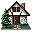Home Existing users log in here or new users sign up here. Nature of Motion Types of Motion Galileo's Pisa Experiment Projectile Motion Juggling

### Motion of a falling object: Quantitative Observational Experiment

#### Aim

Investigate and characterise the motion of a falling object.

#### Prior Knowledge

• Graphing skills.
• Motion diagrams.
• Reference frames.
• Kinematical knowledge: position, velocity.

#### Description of the Experiment

A ball is released from rest. You should take data frame by frame, recording the position of the ball and the time elapsed from a chosen starting point.

Think of the uncertainties in your time and position data.

#### Questions

Decide what co-ordinate system is being used here. Which direction is positive?

1. ##### Analysing the motion
• Do you think the rate at which the ball falls is changing or remaining the same as it falls?

• How can you tell?

• Draw a motion diagram for the ball to aid your discussion.

• Plot a position versus time graph, decide if the falling motion is motion with a constant or changing rate.

• Next, is the rate of motion of the ball changing at a constant rate or a changing rate? How will you answer this question? Consider the following:

• How do you determine the average rate of fall between frames from your data?
• What sort of graph do you obtain? Fit a best fit curve to it if you can.
• What can you say about the change in rate of fall of the ball from the graph?

2. ##### A more accurate determination of the acceleration (advanced analysis).
• Using the position and time data that you have, what sort of graph could you plot in order to determine the acceleration of the ball most accurately?

• Consider the different variables you might choose to get a straight line graph whose slope would yield the acceleration.
• What problems might arise? Consider particularly possible sources of systematic error in your analysis. Where do they come from? Can you eliminate all of them?

• The end result of this discussion is that it is possible to eliminate the systematic errors if you are careful, but it is going to be extremely difficult. It is easier to fit the correct polynomial curve to a graph of position versus time and obtain the acceleration from the best fit curve. This can be done using Excel or your favorite data analysis software.